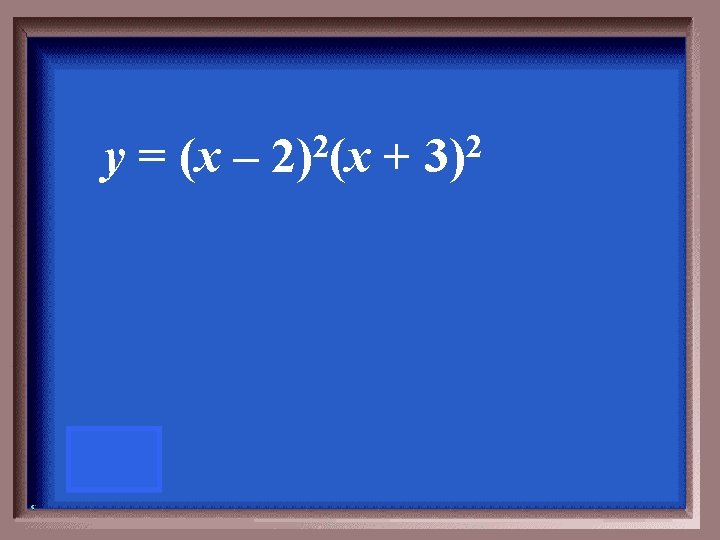# 100 200 200 100 200 300 300 400

• Slides: 74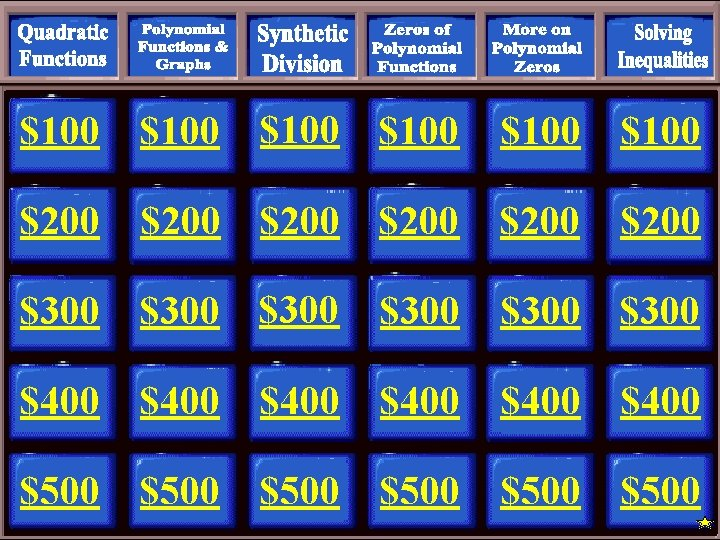\$100 \$200 \$200 \$100 \$200 \$300 \$300 \$400 \$400 \$500 \$500The graph of the equation is shown below.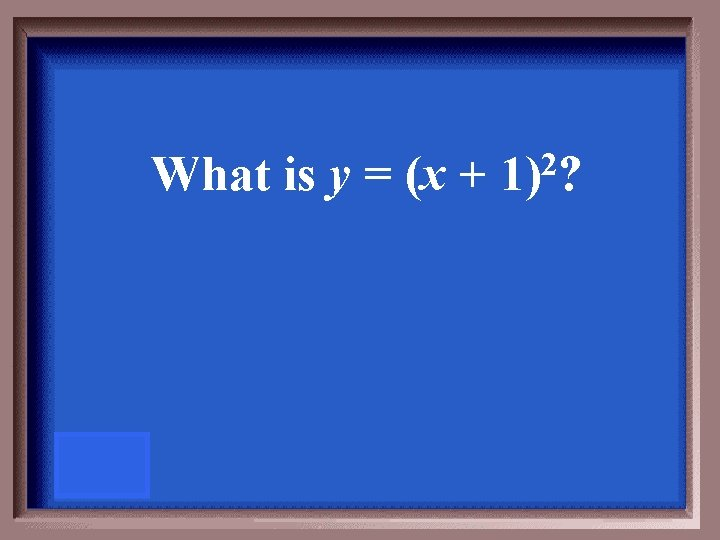What is y = (x + 2 1) ?The equation of the parabola with this vertex is f(x) = (x + 8)2 - 4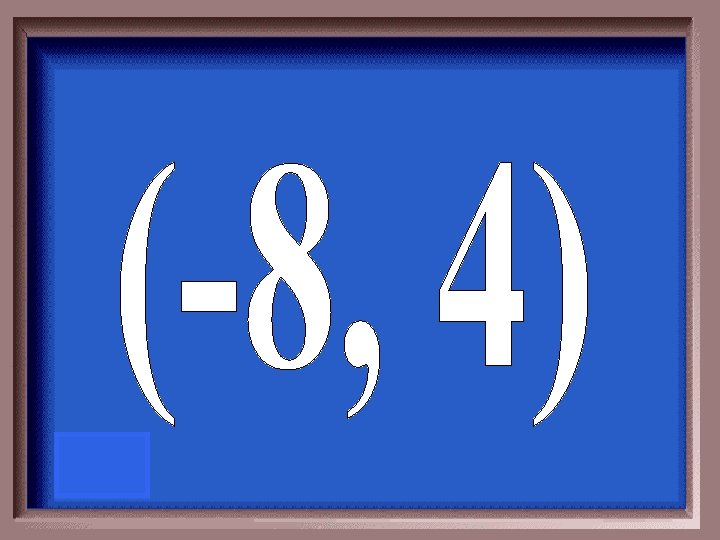The function for this graph is f(x) = (x – 2 5) – 1.What isThis quadratic equation has a maximum point at (3, -4).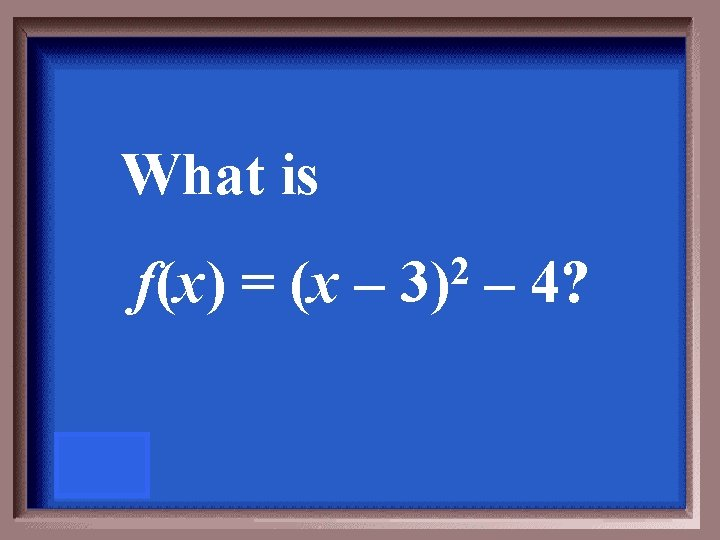What is f(x) = (x – 2 3) – 4?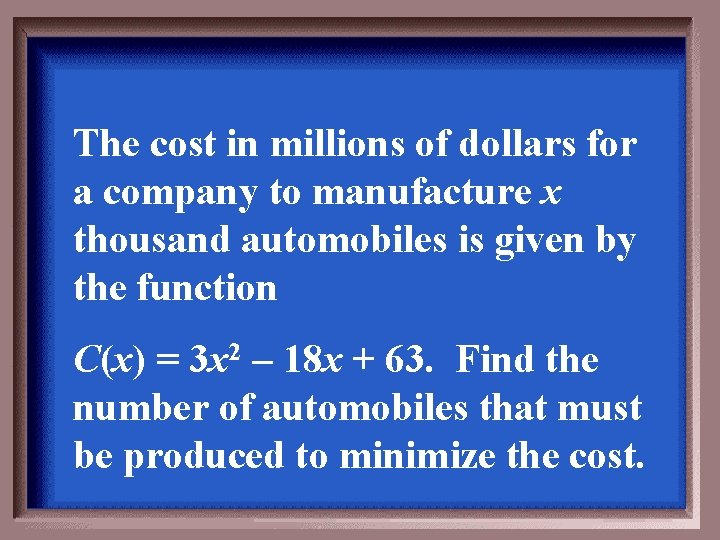The cost in millions of dollars for a company to manufacture x thousand automobiles is given by the function C(x) = 3 x 2 – 18 x + 63. Find the number of automobiles that must be produced to minimize the cost.3 thousand automobilesDetermine if the following is a polynomial function. If so, give the degree. f(x) = x 2 – 3 x 7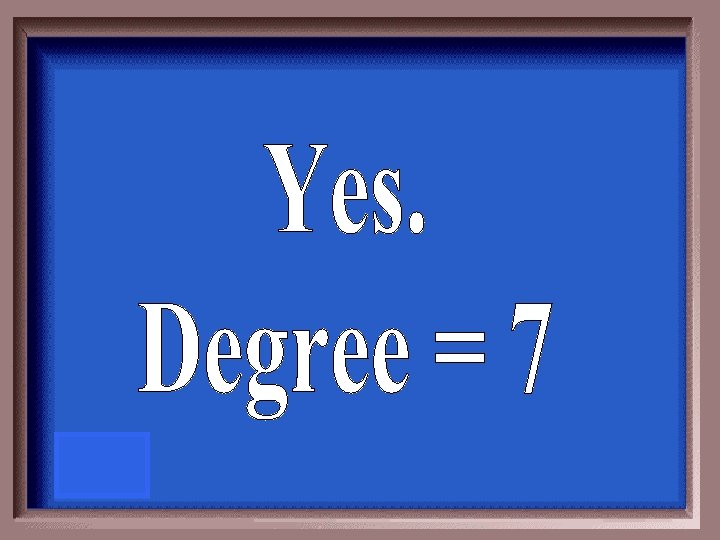Use the leading coefficient test to determine the end behavior f(x) = 6 x 3 + 3 x 2 – 3 x - 1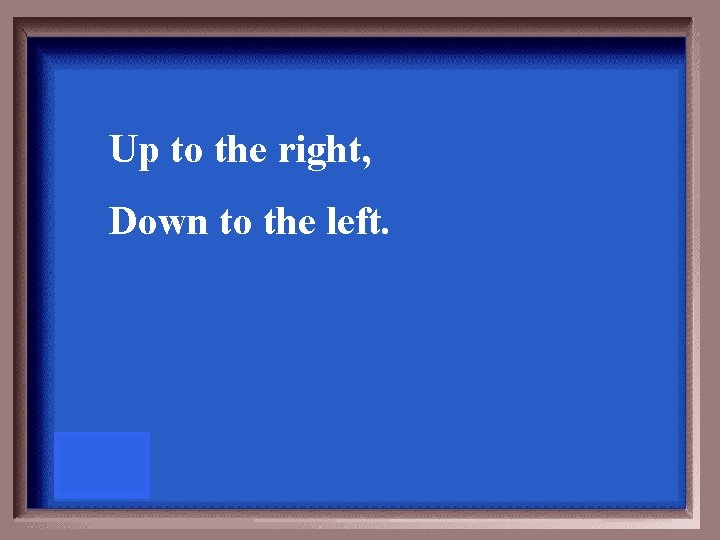Up to the right, Down to the left.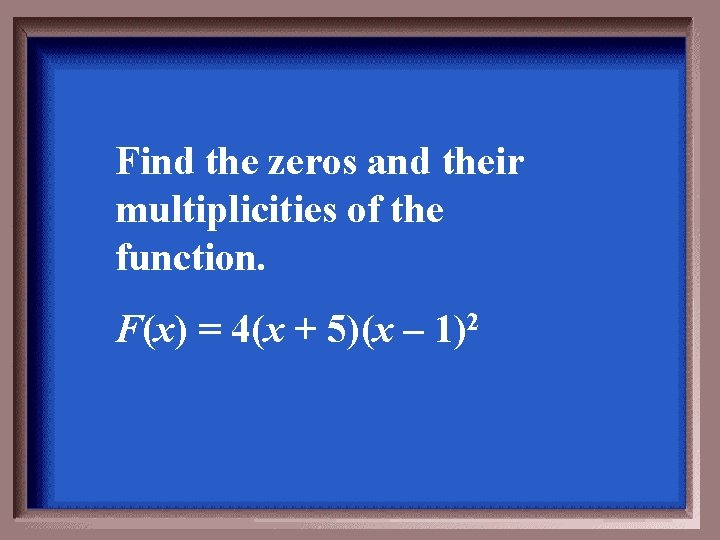Find the zeros and their multiplicities of the function. F(x) = 4(x + 5)(x – 1)2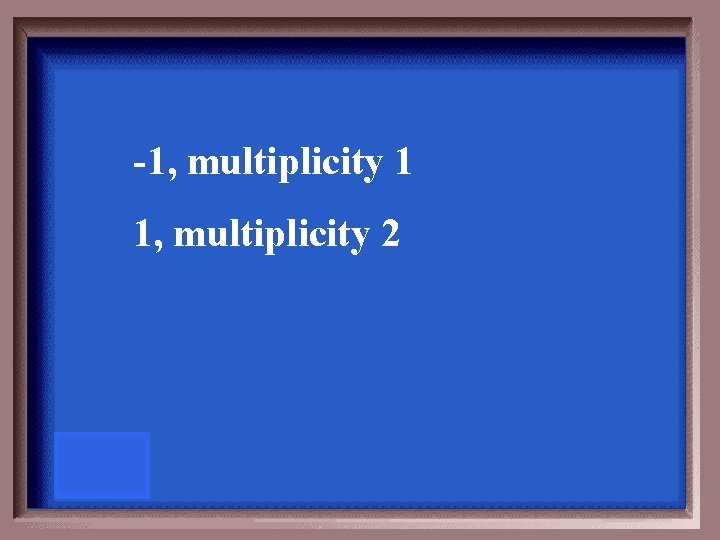-1, multiplicity 1 1, multiplicity 2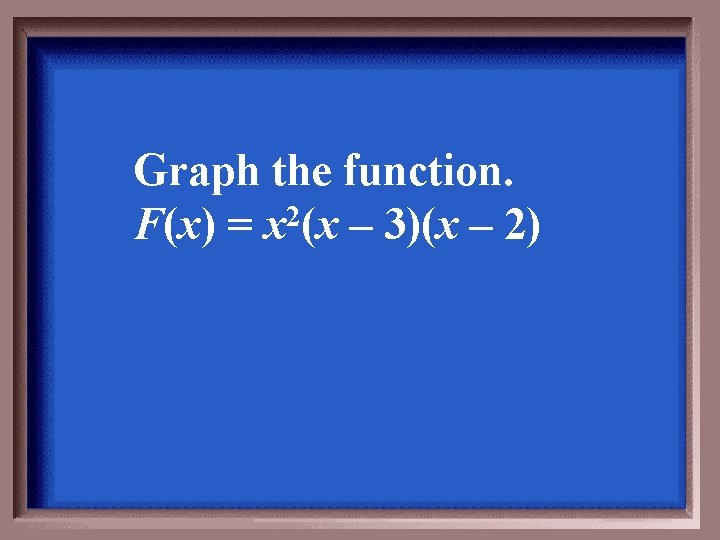Graph the function. F(x) = x 2(x – 3)(x – 2)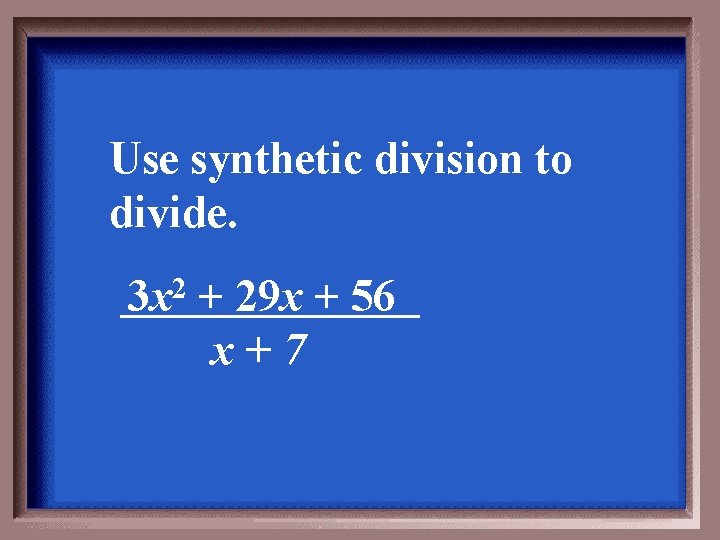Use synthetic division to divide. 3 x 2 + 29 x + 56 x+7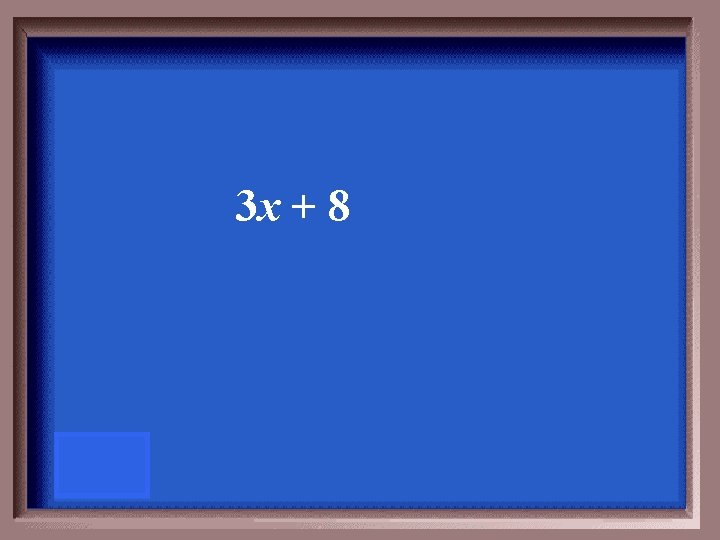3 x + 8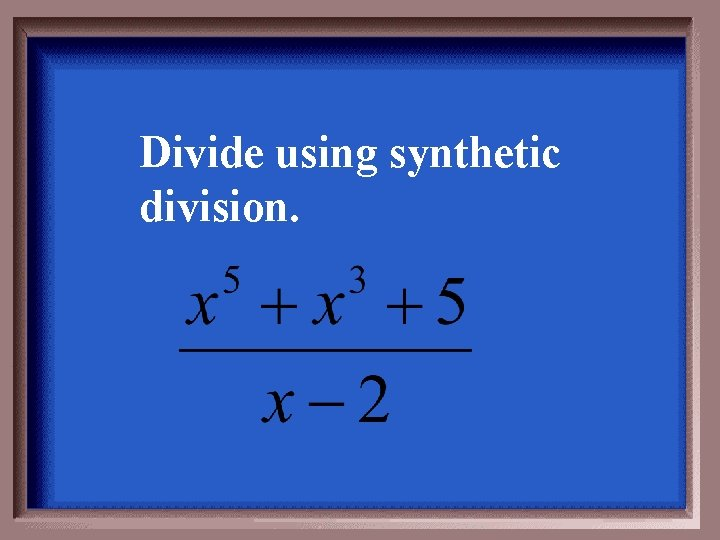Divide using synthetic division.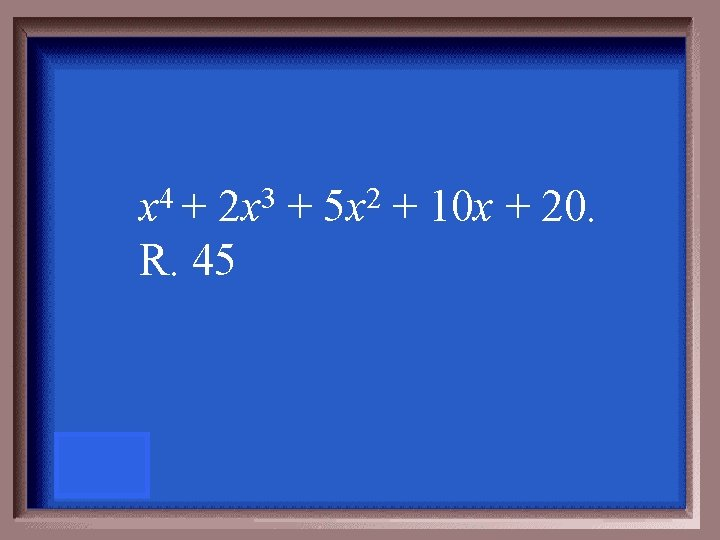4 x + 3 2 x R. 45 + 2 5 x + 10 x + 20.Find f(-3) given f(x) = 4 x 3 – 6 x 2 – 5 x + 6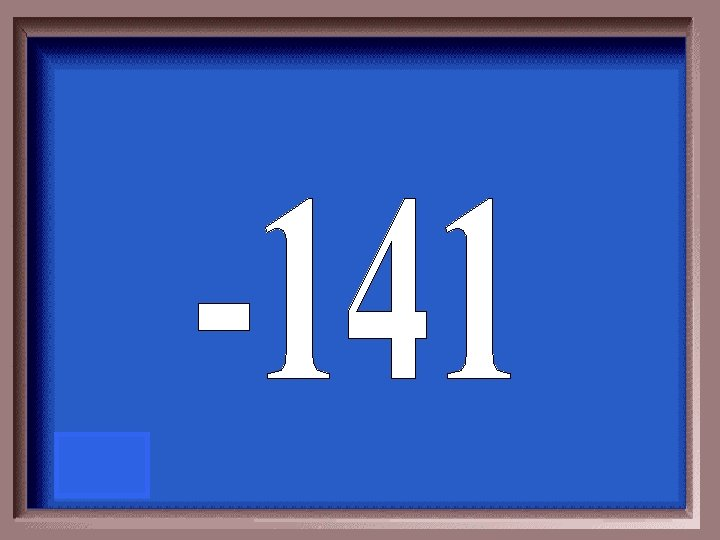Solve the equation 3 x 3 – 28 x 2 + 51 x – 14 = 0 given that 2 is one solution.2, 7, 1/3Use synthetic division to find all zeros of f(x) = 3 x – 2 3 x – 18 x + 40.Use the rational zeros theorem to list all possible rational zeros of f(x) = x 5 – 3 x 2 + 6 x + 14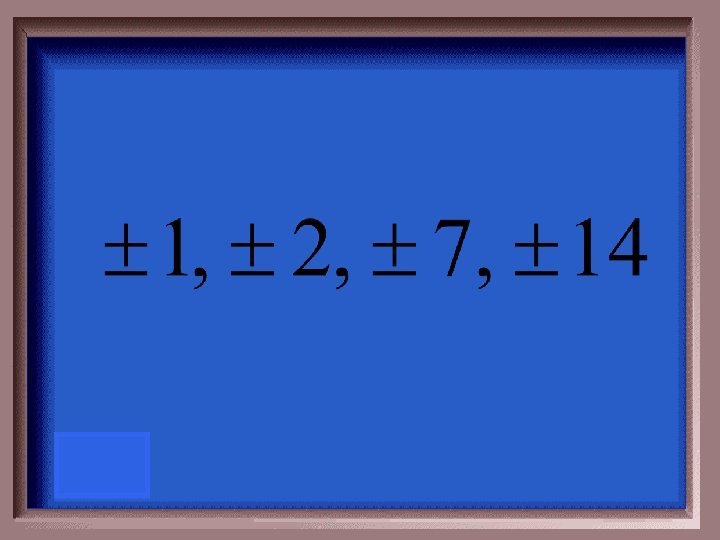Use the rational zeros theorem to list all possible rational zeros of f(x) = 3 3 x – 2 17 x + 18 x + 8 and then use this root to find all zeros of the function.-1/3, 2, 4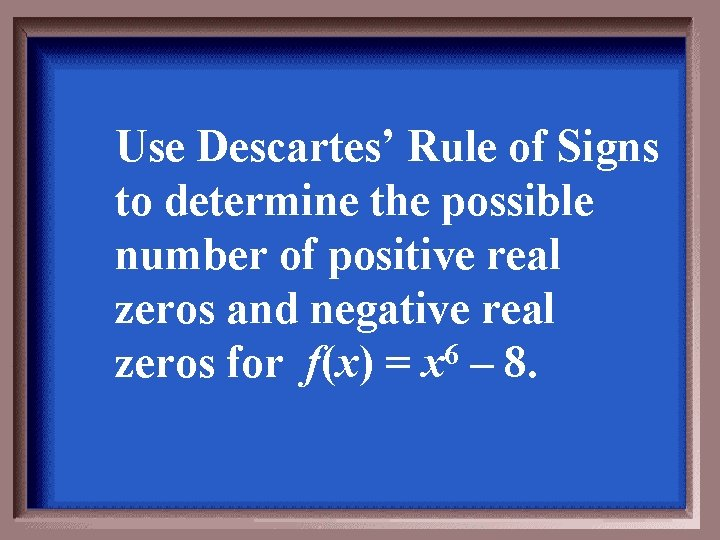Use Descartes’ Rule of Signs to determine the possible number of positive real zeros and negative real 6 zeros for f(x) = x – 8.1 positive real zero 1 negative real zero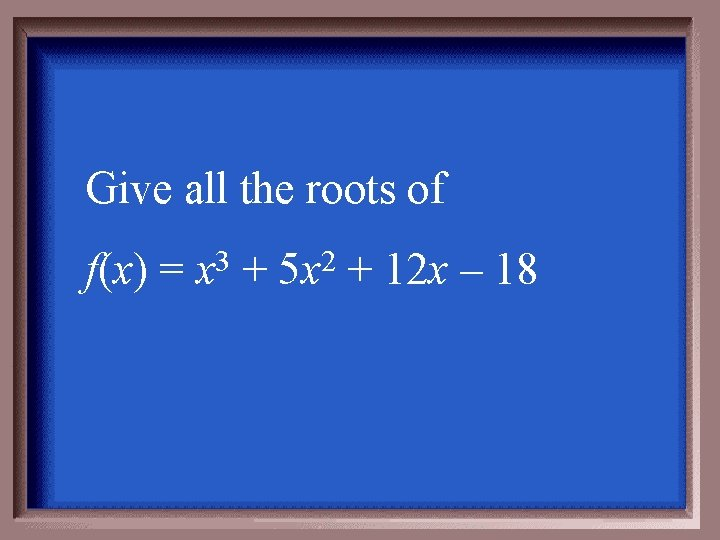Give all the roots of f(x) = 3 x + 2 5 x + 12 x – 18Use the graphing calculator to determine the zeros of f(x) = 3 x – 2 6 x –x+6 1, 3, 4, or 5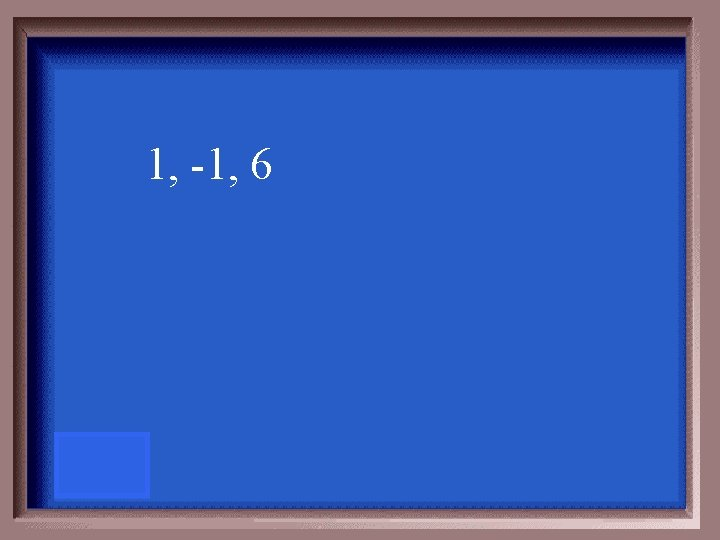1, -1, 6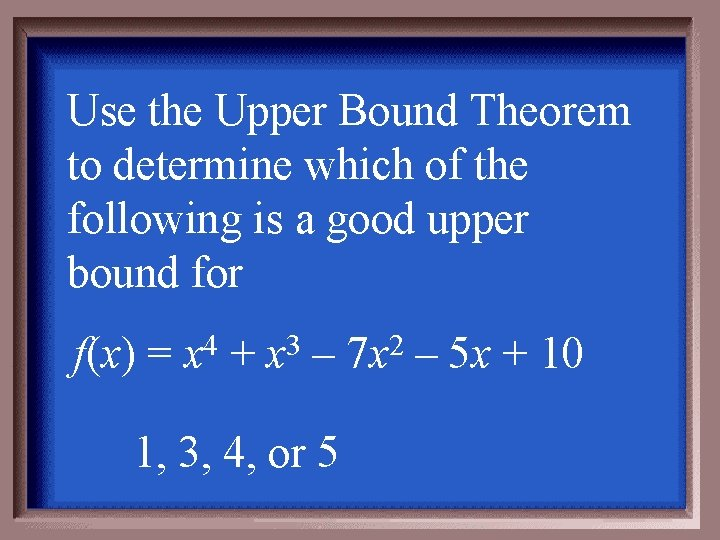Use the Upper Bound Theorem to determine which of the following is a good upper bound for f(x) = 4 x + 3 x – 1, 3, 4, or 5 2 7 x – 5 x + 10Find all roots of the equation. Hint: -2 i is one root. 4 x – 2 21 x – 100 = 0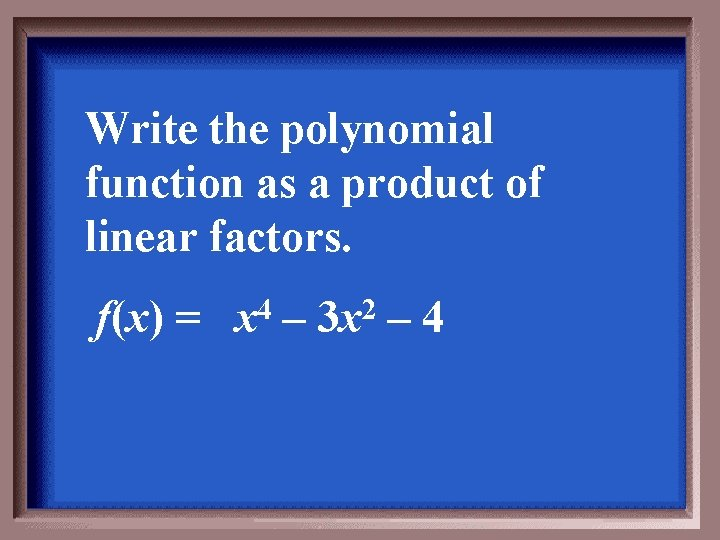Write the polynomial function as a product of linear factors. f(x) = 4 x – 2 3 x – 4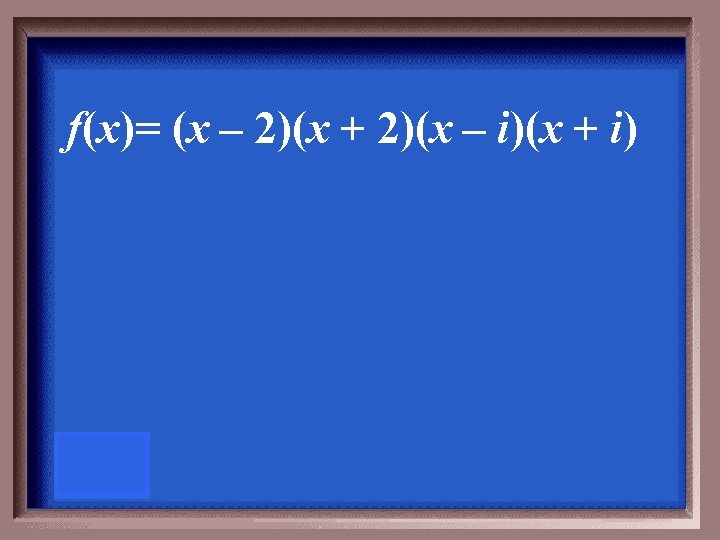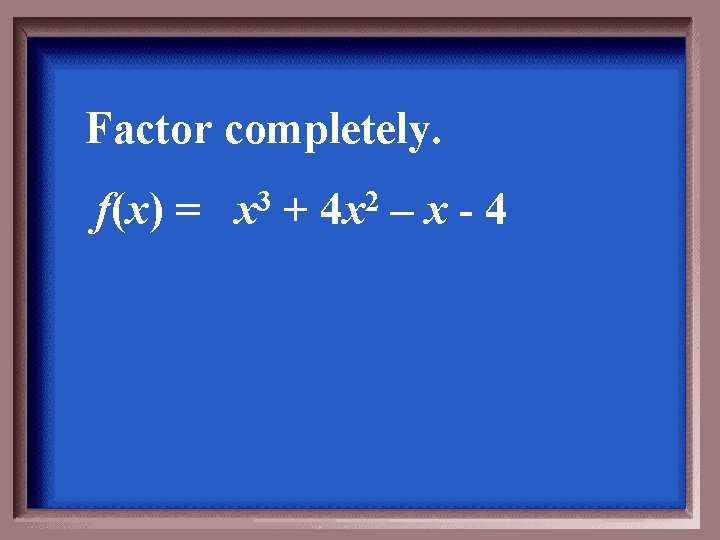Factor completely. f(x) = 3 x + 2 4 x –x-4Give an equation for the polynomial function that has zeros of 2, -2, and 3 and has a degree of 3.f(x)= (x – 2)(x + 2)(x – 3) Other answers are possible.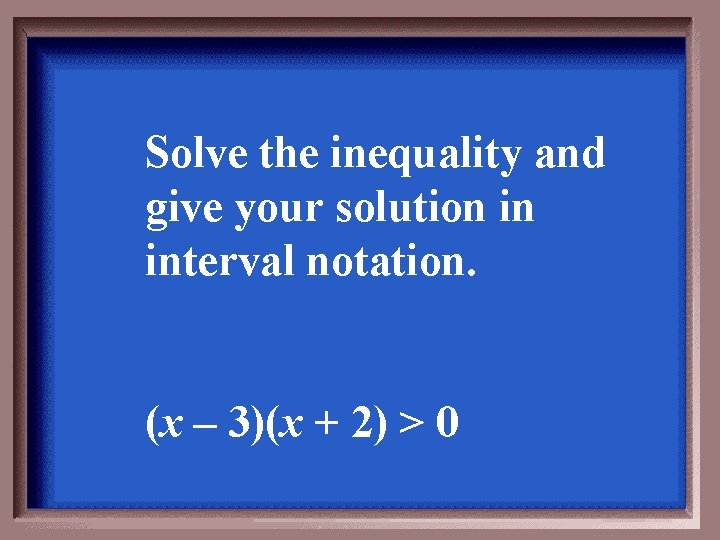Solve the inequality and give your solution in interval notation. (x – 3)(x + 2) > 0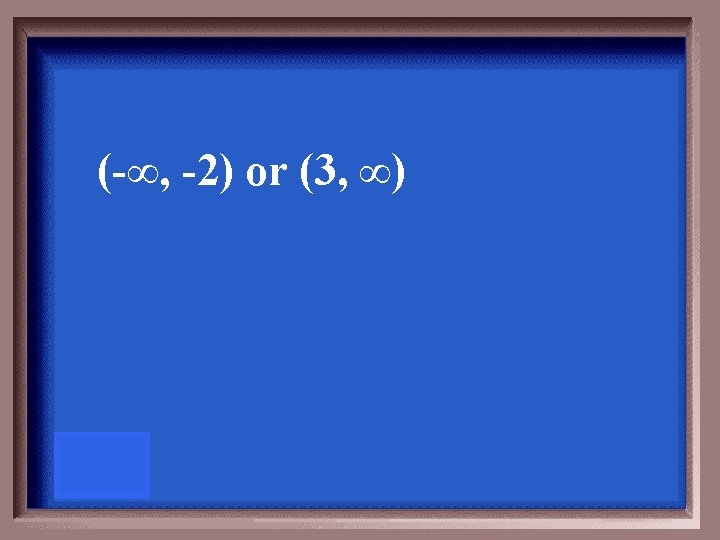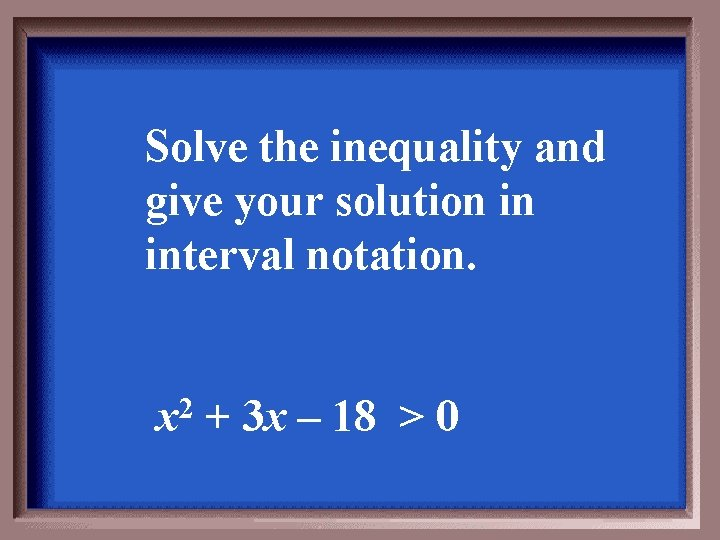Solve the inequality and give your solution in interval notation. x 2 + 3 x – 18 > 0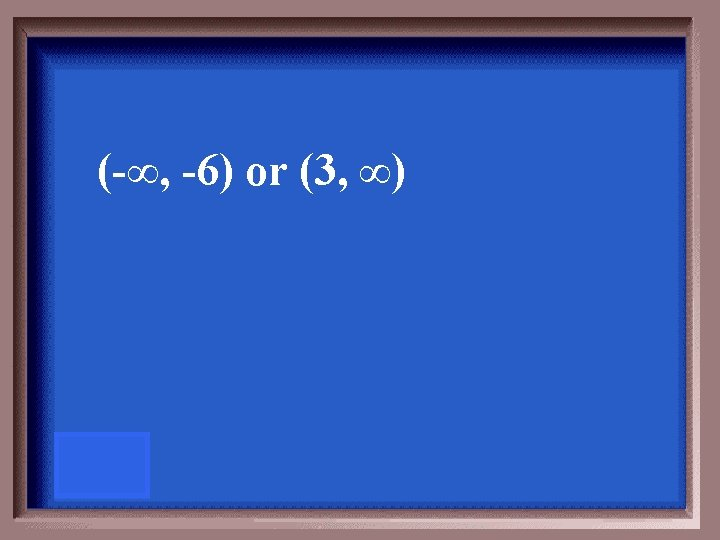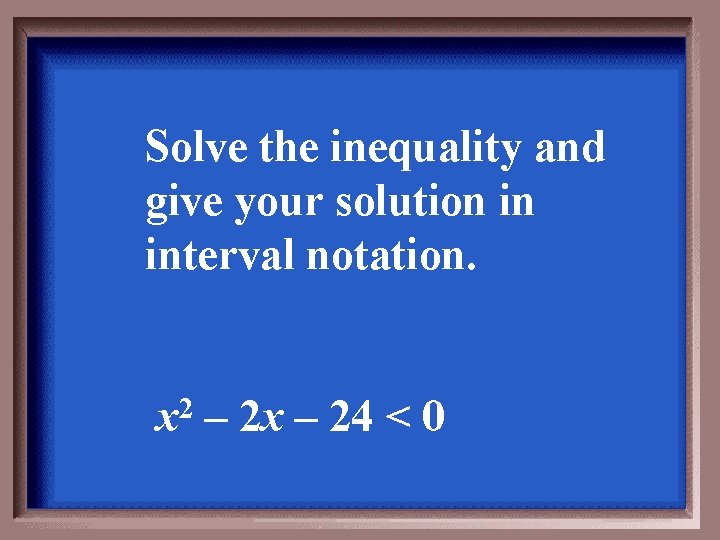Solve the inequality and give your solution in interval notation. x 2 – 2 x – 24 < 0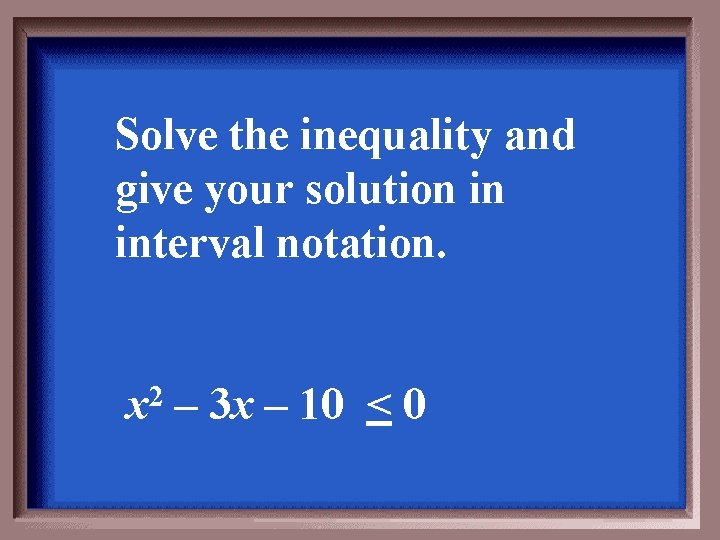Solve the inequality and give your solution in interval notation. x 2 – 3 x – 10 < 0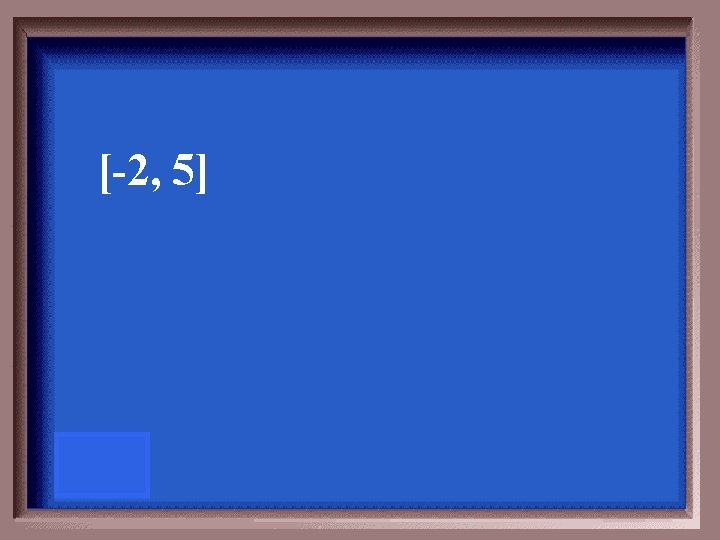Solve the inequality and give your solution in interval notation. x 2 + 6 x < – 8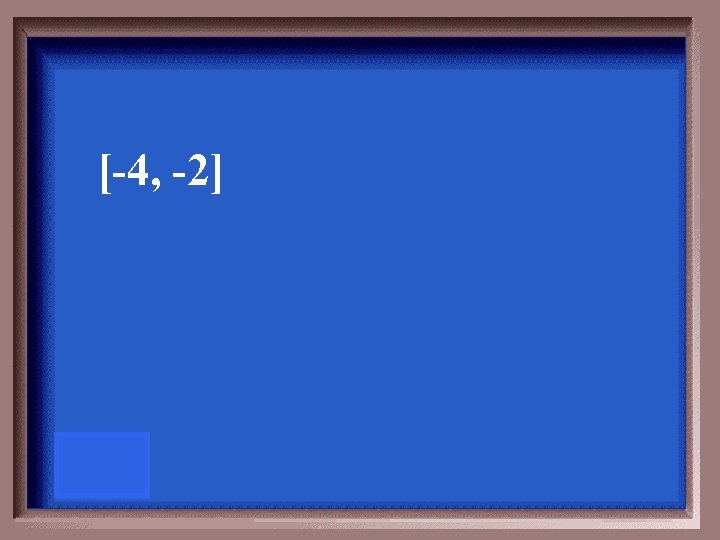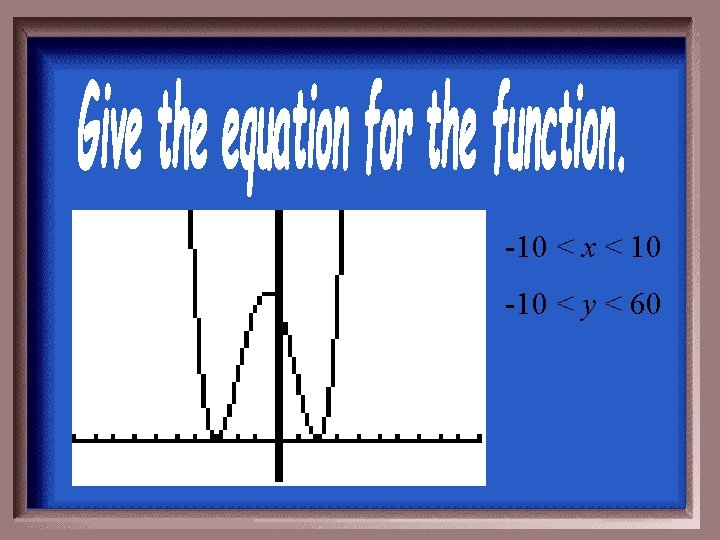-10 < x < 10 -10 < y < 60